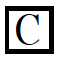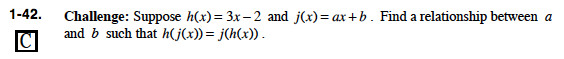### Home > PC > Chapter 1 > Lesson 1.1.3 > Problem1-42

1-42.

Suppose h(x) = 3x − 2 and j(x) = ax + b. Find a relationship between a and b such that h(j(x)) = j(h(x)). Homework Help ✎Manipulate the functions until you have an equation with a and b as the only variables.